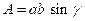Chapter 11.4, Problem 39E### Elementary Geometry for College St...

6th Edition
Daniel C. Alexander + 1 other
ISBN: 9781285195698

#### Solutions

Chapter
Section### Elementary Geometry for College St...

6th Edition
Daniel C. Alexander + 1 other
ISBN: 9781285195698
Textbook Problem
1 views

# Explain why the area of the parallelogram shown is given by the formula(HINT: You will need to use Q N ¯ .)Exercises 41 − 44

To determine

To explain:

The area of the Parallelogram is given by the formula A=absinγ

Explanation

Given,

From the figure,

MN¯=QP¯=b, MQ¯=NP¯=a and M=P=γ

The area of the Parallelogram(A)=The area of the triangle MNQ(A1)+ The area of the triangle QNP(A2)  The area of the triangle MNQ (A1):

For any triangle,

Here, MN¯=b, MQ¯=a and M=γ

The area of the triangle A1=12absinγ

The area of the triangle QNP (A1):

For any triangle,

### Still sussing out bartleby?

Check out a sample textbook solution.

See a sample solution

#### The Solution to Your Study Problems

Bartleby provides explanations to thousands of textbook problems written by our experts, many with advanced degrees!

Get Started